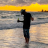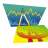# Inertia Question: What exactly is Inertia?

J

#### john3

##### Active member
2019 Donor
Hello,

According to TOS, the inertia plot is the linear regression curve of the Relative Volatility Index (RVI) using the least-square approximation method. TOS plots it as a lower study, but I have seen the code that plots it on an intraday chart.

According to TOS, RVI measures the direction of volatility on a scale from 0 to 100, it is also plotted as a lower indicator.

But I've seen the code that plots inertia on an intraday chart, not as a lower study. Specifically, here is a code: Specifically, here is the code: plot pp = Inertia(hl2(period = AggregationPeriod.DAY)[offset], 252);

Question: Would somebody please explain in plain English what that piece of code does?

Thank you.#### markos

##### Well-known member
VIP
@john3 That appears to be a straight forward linear regression line on a daily aggregation set to display on a 1 year chart. I don't follow the math very well, but, here is a label that shows a linear regression math.

Code:
``````# Linear Regression Math behind Inertia
# TOS Inertia is equivalent
# Mobius
# V01.2011

script LR {
input y = close;
input n = 60;
def x = x + 1;
def a = (n * Sum(x * y, n) - Sum(x, n) * Sum(y, n) ) /
(n * Sum(Sqr(x),n) - Sqr(Sum(x, n)));
def b = (Sum(Sqr(x), n) * Sum(y, n) - Sum(x, n) * Sum(x * y, n) ) /
(n * Sum(Sqr(x), n) - Sqr(Sum(x, n)));
plot InertiaTS = a * x + b;
}
input y = close;
input n = 252;

def lastBar = barNumber();
plot LinReg1 = LR(y, 252);
LinReg1.EnableApproximation();
plot TOS_Inertia = inertia(close, n); # TOS Inertia Function
#TOS_Inertia.SetStyle(Curve.Long_Dash);
#TOS_Inertia.SetLineWeight(3);
#TOS_Inertia.SetDefaultColor(Color.Yellow);
def LinDev = AbsValue(LinReg1 - close);
AddLabel(1, "Linear Deviation N(" + n + ") = " + AsDollars(LinDev) + "  " + AsPercent(LinDev / close), color.white);``````

Last edited by a moderator:
T

#### tomsk

##### Well-known member
VIP
There are actually two very similar sounding TOS library calls used in ThinkScript. Inertia() is TOS method for finding a linear regression curve. On the other hand, inertiaAll() is TOS method for finding a linear regression line

J

#### john3

##### Active member
2019 Donor
Thank you @markos and @tomsk.

plot pp = Inertia(hl2(period = AggregationPeriod.DAY)[offset], 252);

The one above has hl2 in it, so it isn't Inertia() or inertialAll(), which begs the question, what is it?

J

#### john3

##### Active member
2019 Donor
@tomsk So it takes 50% of a prior day's range (an anchor point) and plots a yearly linear regression projection from it?

T

#### TK_44

##### New member
VIP
@john3 That code is just using HL2, or (High + Low)/2, as the data points (as opposed to Close, etc.). It plots a linear regression of the HL2 data point of each bar for the past 252 bars. Think of it like a 252 period moving average using HL2, but instead it is using linear regression. In the case of the code that you posted above it is using a daily aggregation, so the plot will be calculated off of daily bars.

•markos
J

#### john3

##### Active member
2019 Donor
@TK_44 Makes sense now, thank you, I appreciate it.

But, it plots it on an intraday chart, for example, 20 days/15 min. I have always been told that TOS only uses the data visible on a chart. In other words, if I am looking at an intraday chart (20 days, 15 min), it will not plot correctly something that calls for, say, 30 days. Maybe it doesn't apply to a daily aggregation? Because how can it plot 252 days on 20 days?

T

#### TK_44

##### New member
VIP
@john3 It may not apply to daily aggregation, but I’m not for sure. I just say this because you can plot for example an Average Daily Volume with a 30 day look back on a 5 day/5 min chart. I will have to load the study and check it out, but somebody more knowledgeable than me can probably tell you.

•john3My Question is off topic if there is a topic, Does anyone like P H O N K? Questions 0Site Preferences Question Questions 9